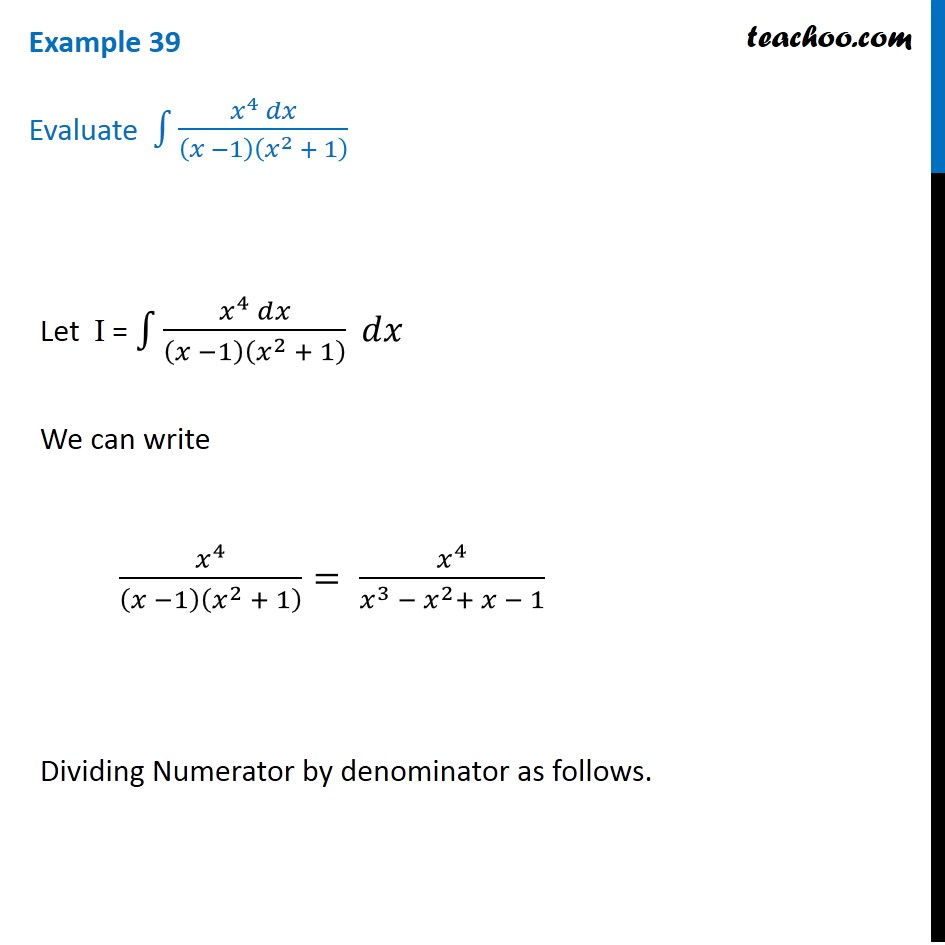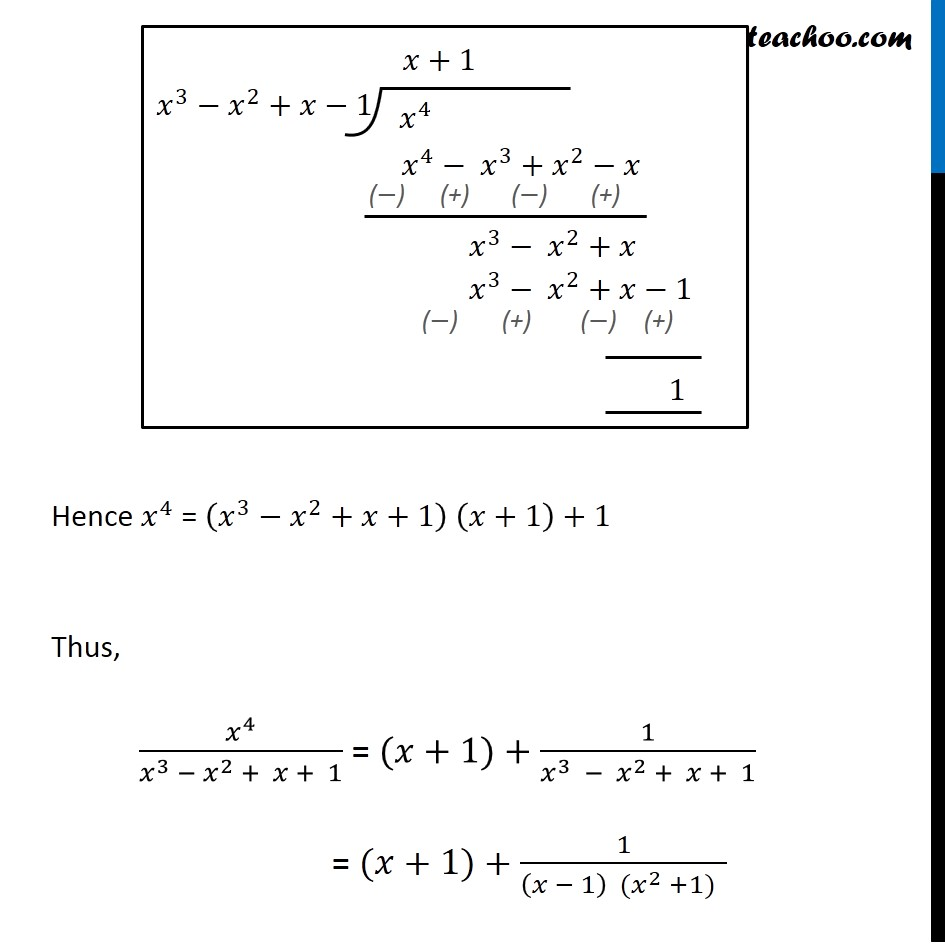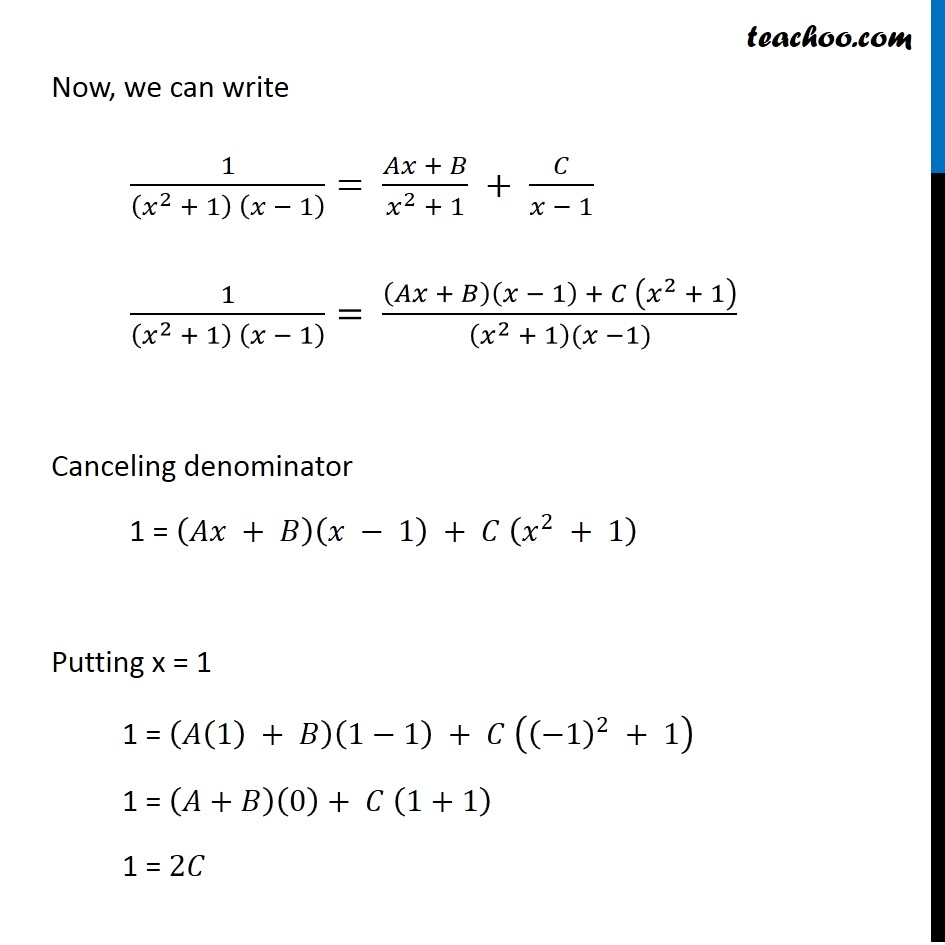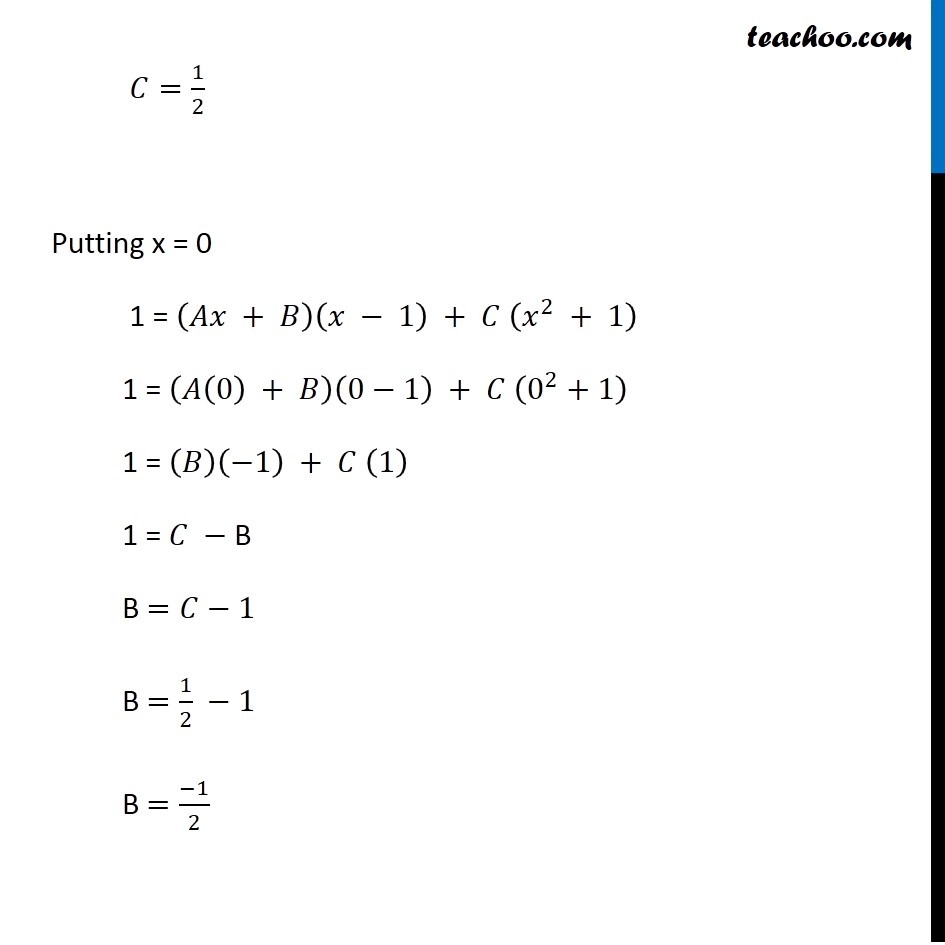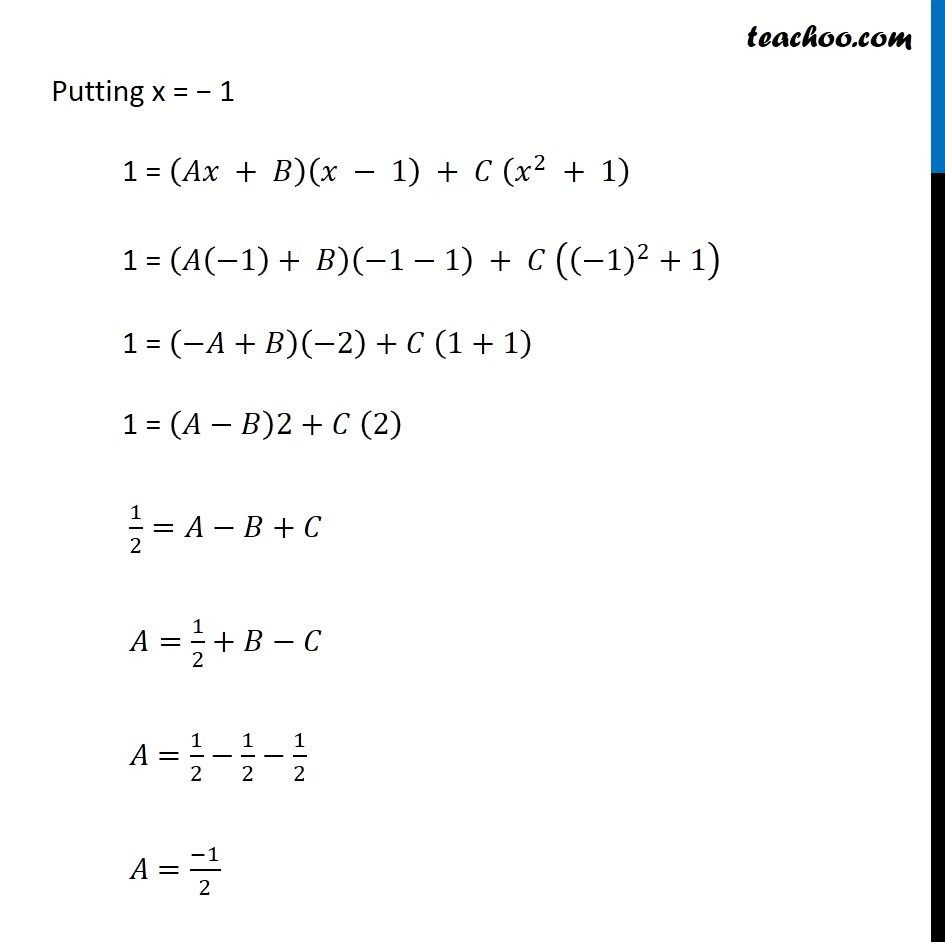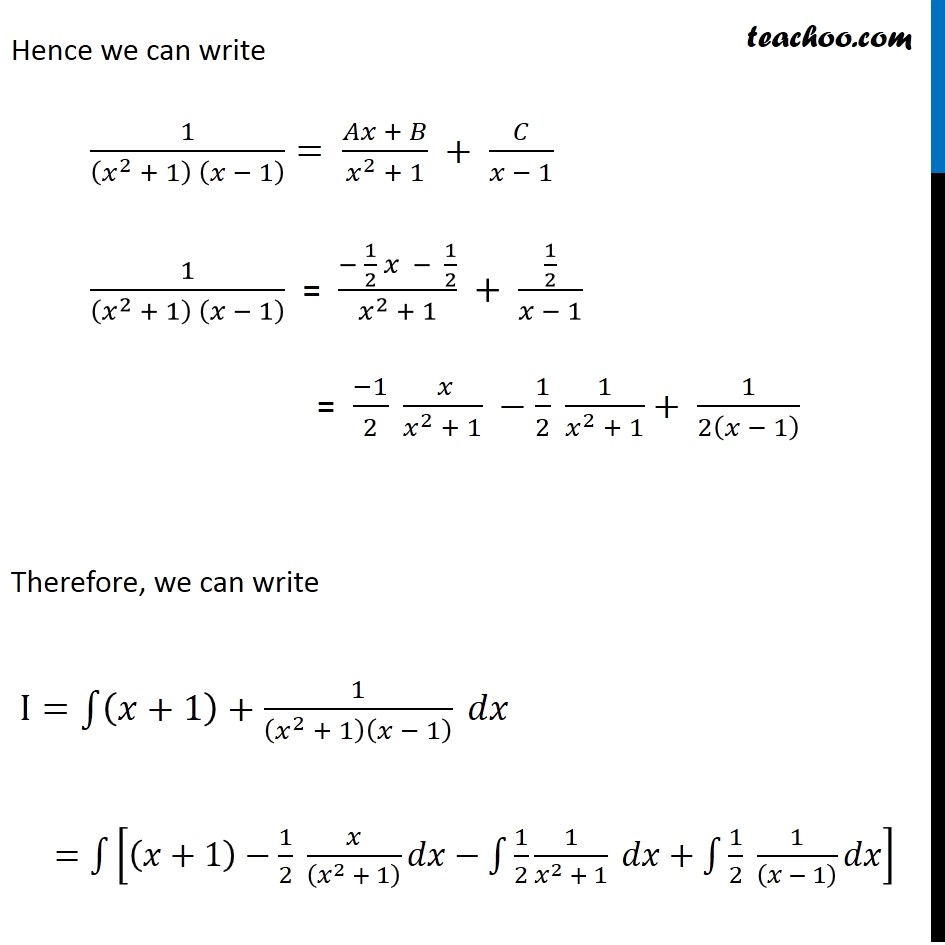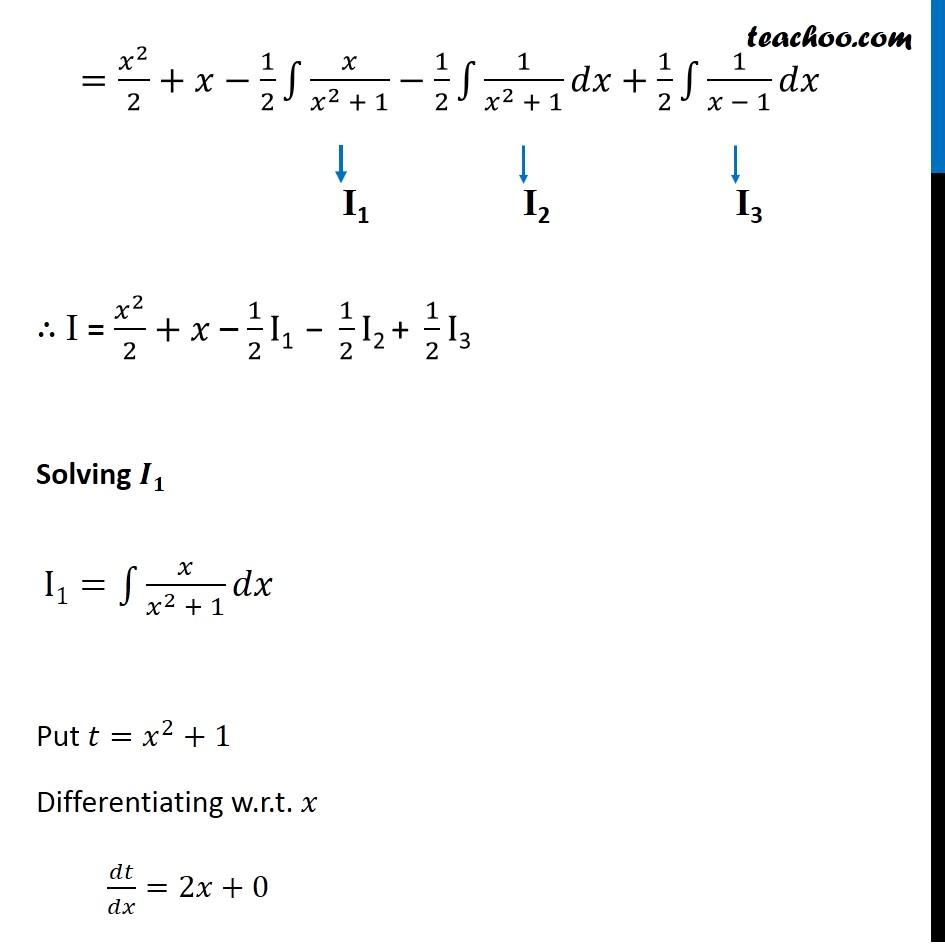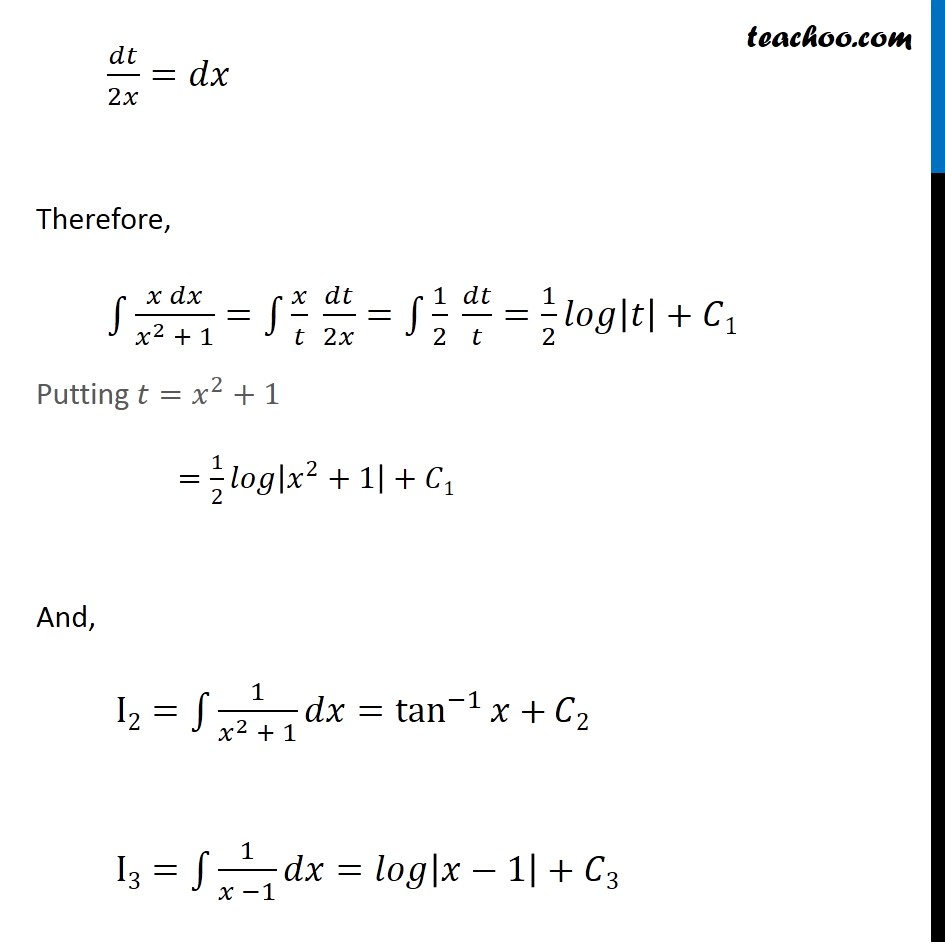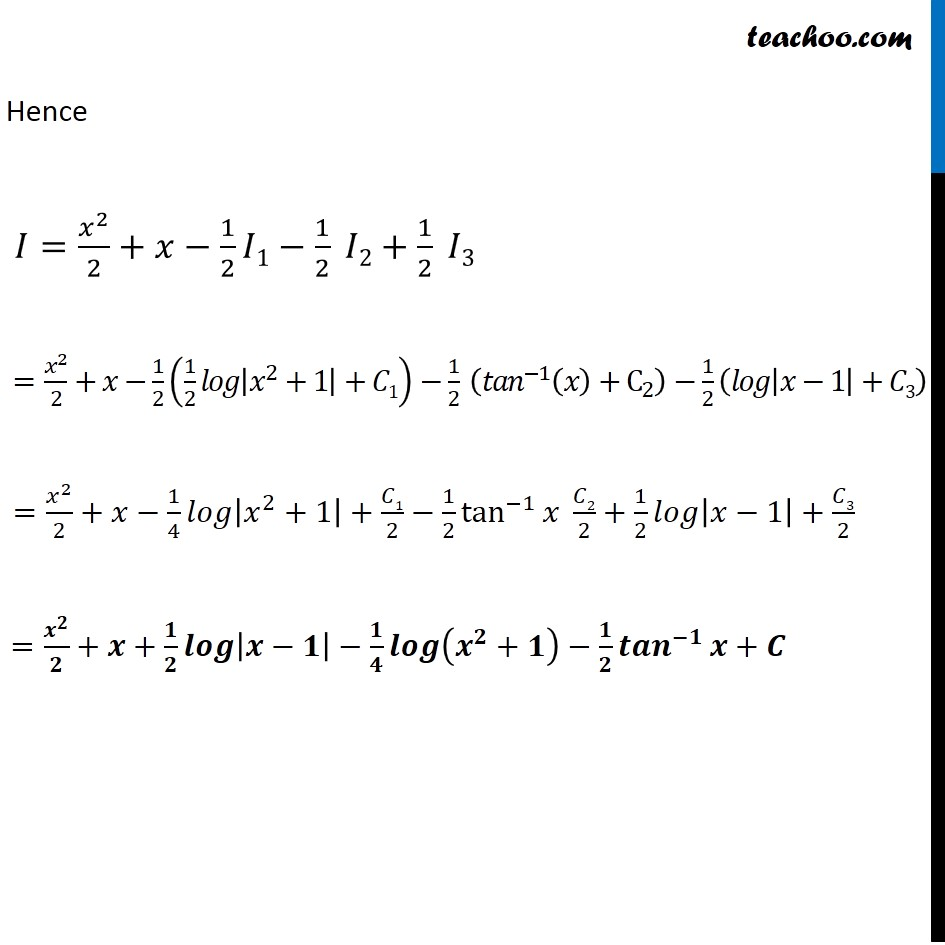1. Chapter 7 Class 12 Integrals (Term 2)
2. Serial order wise
3. Examples

Transcript

Example 39 Evaluate ∫1▒(𝑥^4 𝑑𝑥)/(𝑥 −1)(𝑥^2 + 1) Let I = ∫1▒(𝑥^4 𝑑𝑥)/(𝑥 −1)(𝑥^2 + 1) 𝑑𝑥 We can write 𝑥^4/(𝑥 −1)(𝑥^2 + 1) = 𝑥^4/(𝑥^3 − 𝑥^2+ 𝑥 − 1) Dividing Numerator by denominator as follows. Hence 𝑥^4 = (𝑥^3−𝑥^2+𝑥+1) (𝑥+1)+1 Thus, 𝑥^4/(𝑥^3 − 𝑥^2 + 𝑥 + 1) = (𝑥+1)+1/(𝑥^3 − 𝑥^2 + 𝑥 + 1) = (𝑥+1)+1/((𝑥 − 1) (𝑥^2 +1) ) Now, we can write 1/((𝑥^2 + 1) (𝑥 − 1) )= (𝐴𝑥 + 𝐵)/(𝑥^2 + 1) + 𝐶/(𝑥 − 1) 1/((𝑥^2 + 1) (𝑥 − 1) )= ((𝐴𝑥 + 𝐵)(𝑥 − 1) + 𝐶 (𝑥^2 + 1))/((𝑥^2 + 1)(𝑥 −1)) Canceling denominator 1 = (𝐴𝑥 + 𝐵)(𝑥 − 1) + 𝐶 (𝑥^2 + 1) Putting x = 1 1 = (𝐴(1) + 𝐵)(1−1) + 𝐶 ((−1)^2 + 1) 1 = (𝐴+𝐵)(0)+ 𝐶 (1+1) 1 = 2𝐶 𝐶=1/2 Putting x = 0 1 = (𝐴𝑥 + 𝐵)(𝑥 − 1) + 𝐶 (𝑥^2 + 1) 1 = (𝐴(0) + 𝐵)(0−1) + 𝐶 (0^2+1) 1 = (𝐵)(−1) + 𝐶 (1) 1 = 𝐶 −"B" B =𝐶−1 B =1/2 −1 B =(−1)/2 Putting x = − 1 1 = (𝐴𝑥 + 𝐵)(𝑥 − 1) + 𝐶 (𝑥^2 + 1) 1 = (𝐴(−1)+ 𝐵)(−1−1) + 𝐶 ((−1)^2+1) 1 = (−𝐴+𝐵)(−2)+𝐶 (1+1) 1 = (𝐴−𝐵)2+𝐶 (2) 1/2=𝐴−𝐵+𝐶 𝐴=1/2+𝐵−𝐶 𝐴 =1/2−1/2−1/2 𝐴 =(−1)/2 Hence we can write 1/((𝑥^2 + 1) (𝑥 − 1) )= (𝐴𝑥 + 𝐵)/(𝑥^2 + 1) + 𝐶/(𝑥 − 1) 1/((𝑥^2 + 1) (𝑥 − 1) ) = (− 1/2 𝑥 − 1/2)/(𝑥^2 + 1) + (1/2)/(𝑥 − 1) = (−1)/2 ( 𝑥)/(𝑥^2 + 1) −1/2 1/(𝑥^2 + 1)+ 1/2(𝑥 − 1) Hence we can write 1/((𝑥^2 + 1) (𝑥 − 1) )= (𝐴𝑥 + 𝐵)/(𝑥^2 + 1) + 𝐶/(𝑥 − 1) 1/((𝑥^2 + 1) (𝑥 − 1) ) = (− 1/2 𝑥 − 1/2)/(𝑥^2 + 1) + (1/2)/(𝑥 − 1) = (−1)/2 ( 𝑥)/(𝑥^2 + 1) −1/2 1/(𝑥^2 + 1)+ 1/2(𝑥 − 1) Therefore, we can write I=∫1▒〖(𝑥+1)+1/(𝑥^2 + 1)(𝑥 − 1) 𝑑𝑥〗 =∫1▒[(𝑥+1)−1/2 𝑥/((𝑥^2 + 1) ) 𝑑𝑥−∫1▒〖1/2 1/(𝑥^2 + 1) 𝑑𝑥+∫1▒〖1/2 1/((𝑥 − 1) ) 𝑑𝑥〗〗] =𝑥^2/2+𝑥−1/2 ∫1▒〖𝑥/(𝑥^2 + 1)−1/2 ∫1▒〖1/(𝑥^2 + 1) 𝑑𝑥+1/2 ∫1▒〖1/(𝑥 − 1) 𝑑𝑥〗〗〗 ∴ I = 𝑥^2/2+𝑥 – 1/2 I"1 − " 1/2 I"2 + " 1/2 I"3" Solving 𝑰𝟏 I1=∫1▒〖𝑥/(𝑥^2 + 1) 𝑑𝑥〗 Put 𝑡=𝑥^2+1 Differentiating w.r.t. 𝑥 𝑑𝑡/𝑑𝑥=2𝑥+0 𝑑𝑡/2𝑥=𝑑𝑥 Therefore, ∫1▒〖(𝑥 𝑑𝑥)/(𝑥^2 + 1)=∫1▒𝑥/𝑡 𝑑𝑡/2𝑥〗=∫1▒1/2 𝑑𝑡/𝑡=1/2 𝑙𝑜𝑔|𝑡|+𝐶1 Putting 𝑡=𝑥^2+1 =1/2 𝑙𝑜𝑔|𝑥^2+1|+𝐶1 And, I2=∫1▒〖1/(𝑥^2 + 1) 𝑑𝑥〗=tan^(−1)⁡〖𝑥+〗 𝐶2 I3=∫1▒〖1/(𝑥 −1) 𝑑𝑥〗=𝑙𝑜𝑔|𝑥−1|+𝐶3 Hence 𝐼=𝑥^2/2+𝑥−1/2 𝐼1−1/2 𝐼2+1/2 𝐼3 =𝑥^2/2+𝑥−1/2 (1/2 𝑙𝑜𝑔|𝑥^2+1|+𝐶1)−1/2 (〖𝑡𝑎𝑛〗^(−1) (𝑥)+C_2 )−1/2 (𝑙𝑜𝑔|𝑥−1|+𝐶3) =𝑥^2/2+𝑥−1/4 𝑙𝑜𝑔|𝑥^2+1|+𝐶1/2−1/2 tan^(−1)⁡〖𝑥 𝐶2/2+1/2 𝑙𝑜𝑔|𝑥−1|+𝐶3/2〗 =𝒙^𝟐/𝟐+𝒙+𝟏/𝟐 𝒍𝒐𝒈|𝒙−𝟏|−𝟏/𝟒 𝒍𝒐𝒈(𝒙^𝟐+𝟏)−𝟏/𝟐 〖𝒕𝒂𝒏〗^(−𝟏)⁡〖𝒙+𝑪〗

Examples